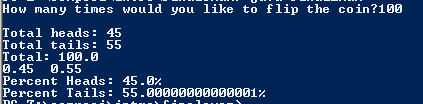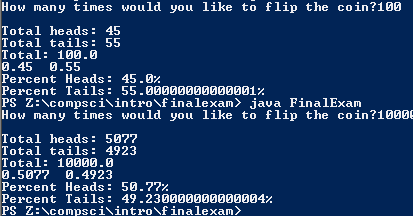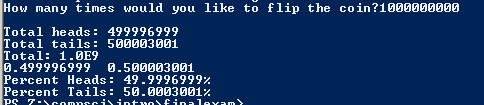# First Semester Final Exam

## Code

```/// Name: Raff Lisbona
/// Period: 6
/// Program Name: Final Exam
/// File Name: FinalExam.java
/// Date Finished: 1/22/2016

import java.util.Scanner;
import java.util.Random;
public class FinalExam
{
public static void main( String[] args )
{
Scanner keyboard = new Scanner(System.in);
Random r = new Random();

int flips, h = 0, t = 0;

System.out.print("How many times would you like to flip the coin?");
flips = keyboard.nextInt();

if ( flips <= 4 || flips > 2100000000 )
{
System.out.println("You can't do that");
System.out.println("How many times would you like to flip the coin?");
flips = keyboard.nextInt();
}
for ( int n = 1; n <= flips; n++ )
{
int flip = r.nextInt(2);
String coin;
if ( flip == 1)
{
coin = "H";
h++;
}
else
{
coin = "T";
t++;
}
}
double total = h + t;
double chanceofH = h / total;
double chanceofT = t / total;
double percentH = chanceofH * 100;
double percentT = chanceofT * 100;